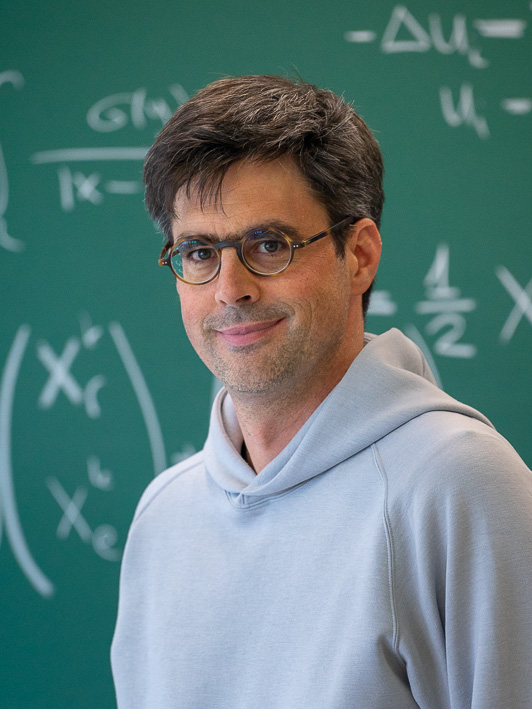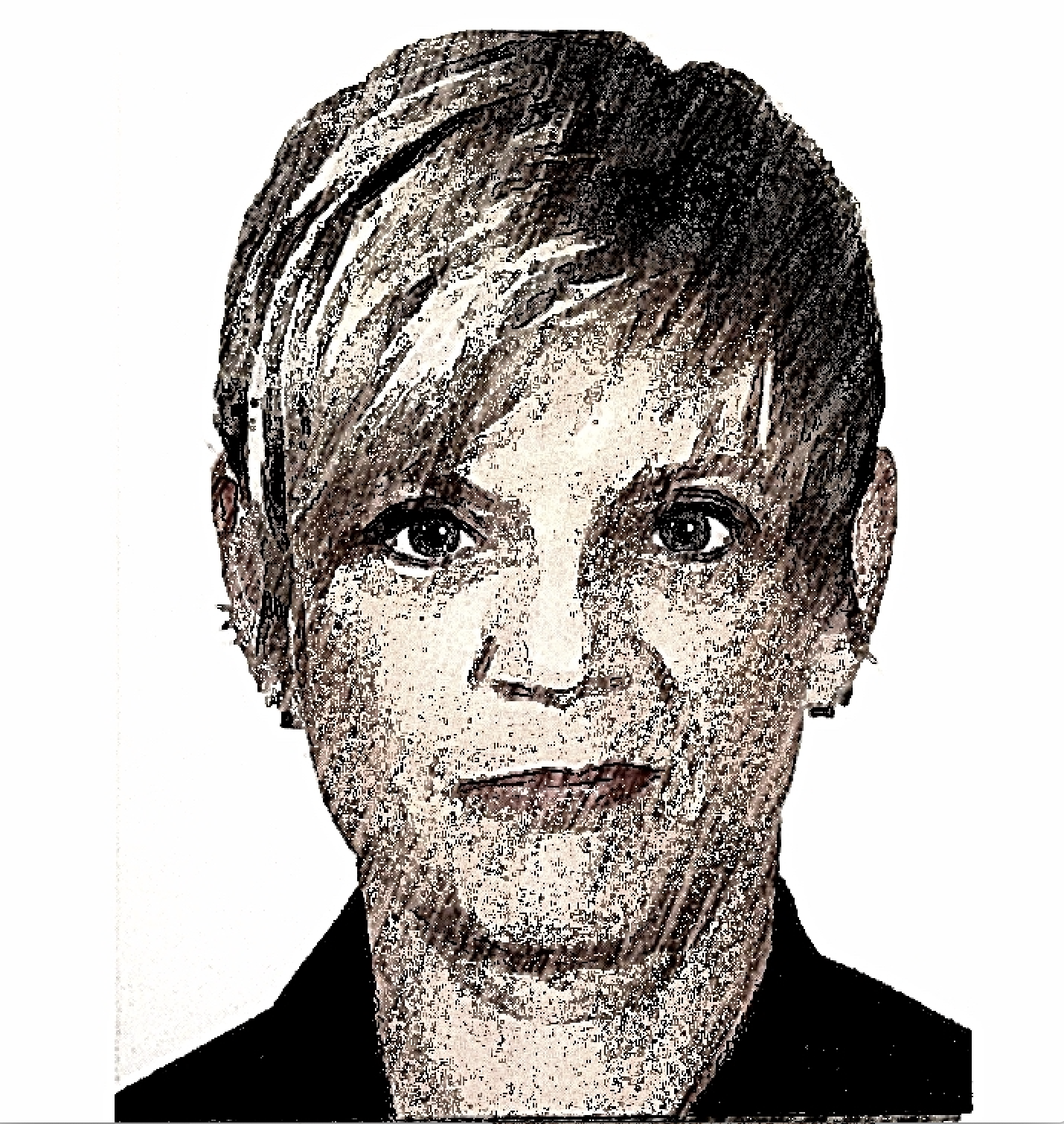# Numerical Mathematics for High Performance Computing

Chair

Efficient discretizations and solvers for partial differential equations (PDE's) and eigenvalue problems

Our research and teaching address a wide range of directions in modern applied mathematics: Mathematical modelling, applied analysis, stochastic techniques and scientific computing provide the theoretical and practical background to understand, predict and optimize highly complex processes in engineering, life, social and natural sciences. Numerical simulation has become the key technology to address these core questions of the University’s scientific and strategic orientation.

Our research involves developing, analyzing and implementing new numerical techniques in both problem-specific and more fundamental settings, and we are equally interested in multidisciplinary applications and theoretical foundations, connecting engineering, natural and computer sciences. We are involved in various national and international collaborations.

In particular, key aspects of our research are efficient numerical methods for the approximation of (non-)linear partial differential equations and eigenvalue problems, and their efficient implementation with applications in computational chemistry and physics.

### Contact### Benjamin Stamm

Prof. Dr.